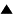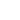Constraint# Constraint

 Constraint is a measure of the reduction of variety or reduction of freedom
A system's variety V measures the number of possible states it can exhibit, and corresponds to the number of independent binary variables. But in general, the variables used to describe a system are neither binary nor independent. For example, if a particular type of berry can, depending on its degree of ripeness, be either small and green or large and red (recognizing only two states of size and color), then the variables "color" and "size" are completely dependent on each other, and the total variety is one bit rather than the two you would get if you would count the variables separately.

More generally, if the actual variety of states that the system can exhibit is smaller than the variety of states we can potentially conceive, then the system is said to be constrained. This means that the system cannot fully use the "freedom" that would seem to be available to it, because some internal or external law, relation or control prohibits certain combinations of values for the variables. Another example of a constrained system is a planet orbiting the sun, which cannot leave its ellipsoid trajectory because of the gravitational force that binds it to the sun, or an atom in a molecule, that can at most vibrate or rotate around its equilibrium position, but cannot get away from the other atoms in the molecule.

Constraint C can be defined as the difference between maximal and actual variety:

C = Vmax - V

Constraint is what reduces our uncertainty about the system's state, and thus allows us to make non-trivial predictions. For example, in the above example if we detect that a berry is small, we can predict that it will also be green. Constraint also allows us to formally model relations, dependencies or couplings between different systems, or aspects of systems. If you model different systems or different aspects or dimensions of one system together, then the joint state space is the Cartesian product of the individual state spaces: S = S1 x S2 x ...Sn. Constraint on this product space can thus represent the mutual dependency between the states of the subspaces, like in the berry example, where the state in the color space determines the state in the size space, and vice versa.

Variety and its complement, constraint, can be generalized to a probabilistic framework, where they are replaced respectively by entropy and information.

 HomeMetasystem Transition TheorySystems Concepts Up Prev.Next Down